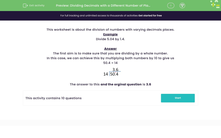# Divide Decimals with a Varying Number of Decimal Places

In this worksheet, students will carry out divisions of numbers with varying numbers of decimal places.Key stage:  KS 3

Curriculum topic:   Number

Curriculum subtopic:   Use Four Operations for All Numbers

Popular topics:   Fractions worksheets, Percentages worksheets, Decimals worksheets

Difficulty level:#### Worksheet Overview

This activity is about the division of numbers with varying decimal places.

Example

Divide 5.04 by 1.4.

The first aim is to make sure that you are dividing by a whole number.

In this case, we can achieve this by multiplying both numbers by 10 to give us

50.4 ÷ 14The answer to this and the original question is 3.6

Remember that you must multiply both the numbers by the same amount or the answer will be wrong!

Let's practise this in the questions that follow.

### What is EdPlace?

We're your National Curriculum aligned online education content provider helping each child succeed in English, maths and science from year 1 to GCSE. With an EdPlace account you’ll be able to track and measure progress, helping each child achieve their best. We build confidence and attainment by personalising each child’s learning at a level that suits them.

Get started